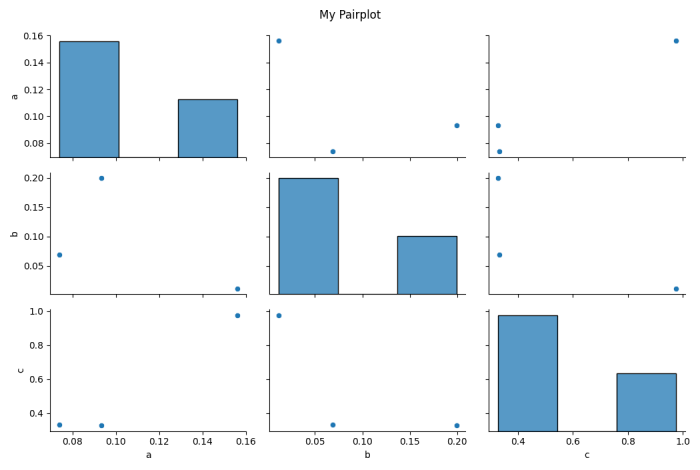# How to show the title for the diagram of Seaborn pairplot() or PridGrid()? (Matplotlib)

To show the title for the diagram for Seaborn pairplot(), we can use pp.fig.suptitle() method.

## Steps

• Set the figure size and adjust the padding between and around the subplots.
• Create a Pandas dataframe, i.e., a two-dimensional, size-mutable, potentially heterogeneous tabular data.
• Plot pairwise relationships in a dataset.
• Add a centered title to the figure.
• To display the figure, use show() method.

## Example

import seaborn as sns
import pandas as pd
import numpy as np
import matplotlib.pyplot as plt

plt.rcParams["figure.figsize"] = [7.50, 3.50]
plt.rcParams["figure.autolayout"] = True

df = pd.DataFrame(np.random.random((5, 5)),
columns=["a", "b", "c", "d", "e"])

pp = sns.pairplot(df, height=3.5)
pp.fig.suptitle("My Pairplot")

plt.show()

## Output